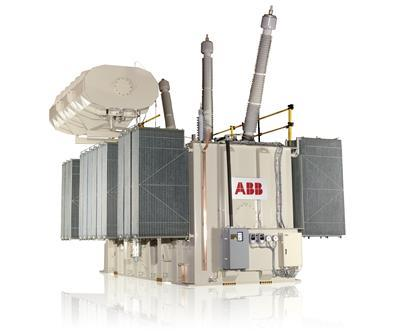News

Is the ratio of the three-phase transformer the ratio of the phase voltage to the line voltage?

Regardless of the transformer, the ratio is equal to the ratio of the turns of the coil, and the ratio of the turns of the coil is equal to the ratio of the phase voltage. That is to say, the transformation ratio of the three-phase transformer is the ratio of the phase voltages.

For power systems, because the line is approximately three-phase symmetrical, the line voltage ratio is considered to be equal to the phase voltage ratio. Therefore, for the sake of convenience, the ratio of the three-phase transformer is generally written as the ratio of the line voltage. However, if the line is asymmetrical, the line voltage ratio will not be equal to the phase voltage ratio.

The definition of the three-phase transformer ratio: For a three-phase transformer, the ratio refers to the ratio of the primary and secondary phase electromotive forces, that is, the ratio of the primary and secondary phase voltages.